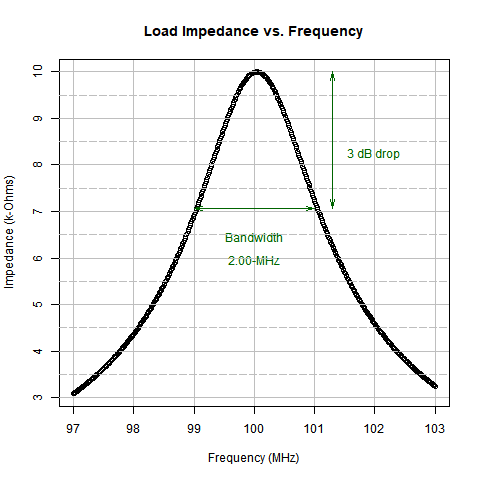# Impedance Transformer Design Tool

Marcel Merchat
RF and Microwave Engineer

### Reproducible Report

#### This slideshow is also an engineering tool. It is produced by a computer script program. Nothing is performed manually as all calulations are performed in code blocks that are mostly hidden. You can spot visible code blocks by rectangular bounded blocks like this one.

pi

##  3.141593


## Part A: Transformer with Default Bandwidth

### Simple Matching Transformer with Default Bandwidth

RF Small Signal AC Model Amplifier Load Impedance (RL)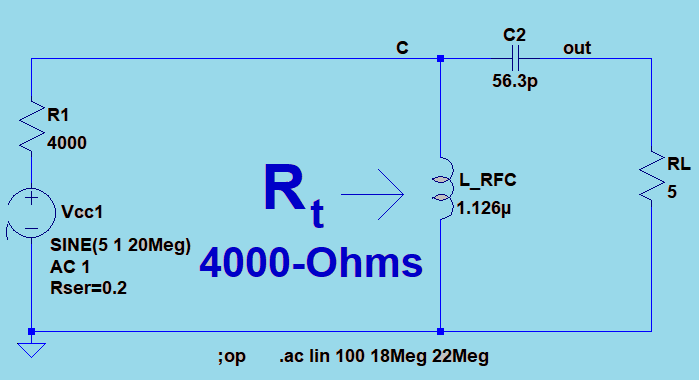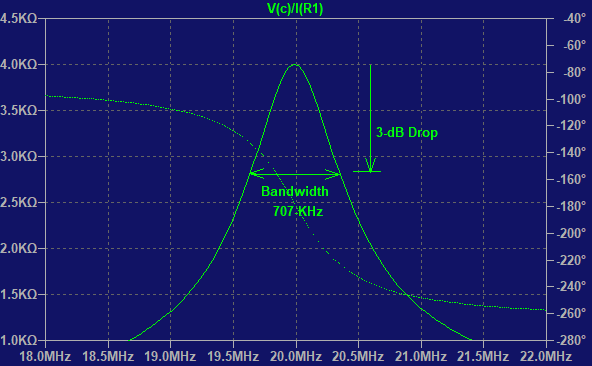This parallel resonant circuit presents a matching impedance of 4-k$\Omega$ at 20 MHz to the power amplifier. The 5-$\Omega$ resistor is transformed to 4-k$\Omega$ in this manner.

The transformed load impedance Rt has a peak at 20-MHz where it matches the source resistance of 4-k$\Omega$. The bandwidth of 707-kHz is the only one possible for this simple circuit.

### Circuit Q

#### We will use a concept called Q which represents the relative circuit bandwidth. Let $f_o$ be the resonant frequency of the amplifier and let $f_1$ and $f_2$ be the upper and lower limits of the tuned bandwidth of the transformer. We set the transmitter frequency to $fo=20$ MHz and define Q as follows:

$Q=\frac {f_o}{f_2-f_1}$

$B=\frac {f_o} {Q}$

### Part A: Simple Circuit

#### This method provides a defined bandwidth that cannot be adjusted. We begin with a formula from page 44 of the textbook we reference at the end of the slideshow.

$R_t=(1+Q^2) \cdot R_L$

### Design Calulations without Bandwidth Adjustment

#### If the power amplifier has an internal output impedance of 4-k$\Omega$ and a load $R_L$ of 5-$\Omega$, the parallel resonant circuit must transform the 5-$\Omega$ resistance to 4-k$\Omega$. We will design the matching transformer as follows. We calculate Q = 28.3.

formatC(sqrt(Rt/RL-1), digits = 1, format = "f")

##  "28.3"


### Design Calulations for Unadjusted Bandwidth ... continued

#### The bandwidth is already fixed for this design before any component values have been calculated. We calculate the bandwidth as B = 707-kHz using our concept of Q as follows; the extra factor of 1000 converts the result to kHz. Paste is a string operation that displays the result with the kHz units.

##### $B= \frac {f_o}{Q}$
paste(formatC(fo/(Q1*1000), digits = 1, format = "f"), "-kHz", sep="")

##  "707.5-kHz"


### Determine Capacitor

##### $C = \frac {1}{\omega \cdot X_C}$
## branch impedance X_c:
paste(formatC(Q * RL, digits = 1, format = "f"), "-Ohms", sep="")

##  "141.3-Ohms"

## branch capacitance C:
paste(formatC((1/ (w * Xc)) * 10^12, digits = 1, format = "f"), "-pF", sep="")

##  "56.3-pF"


### Determine Inductor

###### $L= \frac {1}{\omega \cdot X_C}$
## Branch inductance L:
paste(formatC(( Rt/(Q * w)) * 10^6 , digits = 3, format = "f"), "-uH", sep="")

##  "1.126-uH"


### Netlist

##   " * C:/Users/merch/OneDrive/Documents/LTSpice/RF/impedanceTransformers/imp_transfx/RF_impedance_Transformer_NoBW.asc"
##   "L_RFC c 0 1.12609942741738e-06 Ipk=12 Rser=0.0 Cpar=0"
##   "C1 c out 5.63049713708692e-11 V=2.5K Irms=0 Rser=0 Lser=0"
##   "RL out 0 5"
##   "Rt N01 c  4000"
##   "Vcc N01 0 SINE(018.921.1Meg) AC 1 Rser=0"
##   ";op"
##   ".ac lin 500 18.9Meg 21.1Meg"
##   ".backanno"
##  ".end"
##  "NA"
##  ""
##  ""


Impedance Response for simple Circuit with Default Bandwidth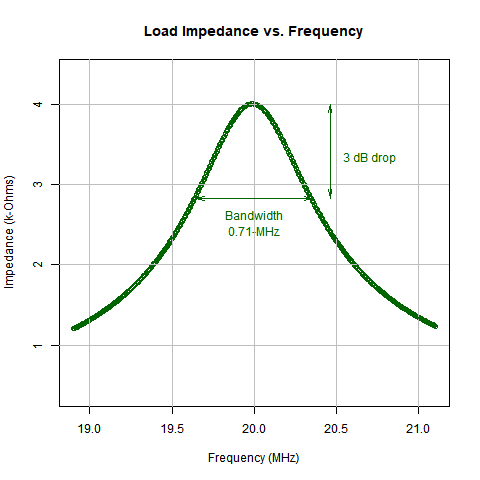The response of the transformer has a peak at 20-MHz where it matches the source resistance of 4-k$\Omega$ for the power amplifier. The 707-kHz bandwidth is the only one possible for this simple circuit.

## Part B: Transformer with Designed Bandwidth

#### Load impedance $RL$ = 1-$k\Omega$

Small Signal Model for 2-MHz Bandwidth at 100-MHz.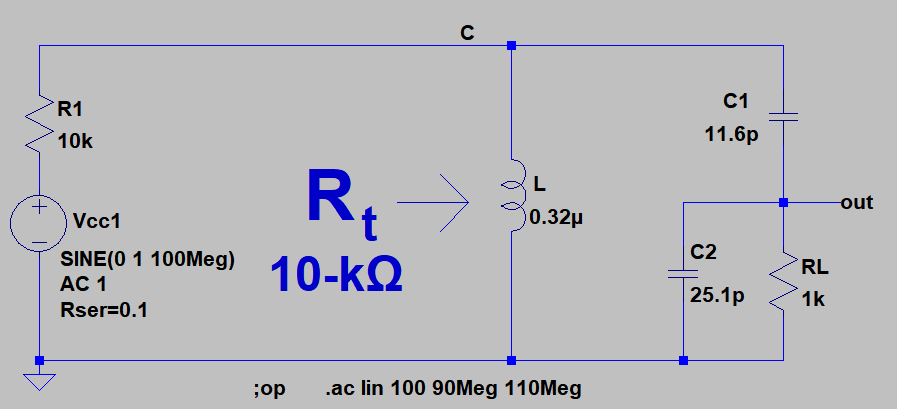#### Step-1: Circuit Q for Bandwidth Control

###### $Q_t= \frac {f_o}{BW}$
paste("Qt =", formatC(Qt, digits = 1, format = "f"))

##  "Qt = 50.0"

###### $Q_p= \sqrt{\frac {R_L}{R_t} \cdot (Q_t^2 + 1) -1}$
paste("Qp =", formatC(sqrt((RL/Rt) * (Qt^2 + 1) - 1), digits = 2, format = "f"))

##  "Qp = 15.78"


#### Step-2: Calculate Capacitance of C2

###### C2 = $\sqrt{\frac {Q_p}{w \cdot R_L}}$
paste("C2 = ", formatC((Qp/(w * RL)) * 10^12, digits = 1, format = "f"), "-pF", sep="")

##  "C2 = 25.1-pF"


#### The parallel tap components C2 and RL are replaced by the series combination of $C_{se}$ and $R_{se}$ respectively.

##### $C_{se} = C_2 \cdot (\frac {1}{Q_p^2+1})$
paste("Cse = ", formatC((C2 * (1/(Qp^2) + 1)) * 10^12, digits = 1, format = "f"), "-pF", sep="")

##  "Cse = 25.2-pF"

##### $R_{se} = \frac {RL}{Q_p^2+1}$
paste("Rse = ", formatC(RL/((Qp^2) + 1), digits = 1, format = "f"), "-Ohms", sep="")

##  "Rse = 4.0-Ohms"

##### $X_C = Q_t \cdot R_{se}$
paste("XC = ", formatC(XC, digits = 1, format = "f"), "-Ohms", sep="")

##  "XC = 199.9-Ohms"

paste("C = ", formatC((1/(w*XC)) * 10^12, digits = 1, format = "f"), "-pF", sep="")

##  "C = 8.0-pF"

##### $C1= \frac {-C \cdot C_{se}}{C - Cse}$
paste("C1 = ", formatC((-Cse * C / (C - Cse)) * 10^12, digits = 1, format = "f"), "-pF", sep="")

##  "C1 = 11.6-pF"


### Determine Inductor

###### Solve this quadratic for L at resonant frequency $w$
paste(formatC(L * 10^6, digits = 2, format = "f"), "-uH", sep="")

##  "0.32-uH"


### Netlist

##   " *  C:/Users/merch/OneDrive/Documents/LTSpice/RF/impedanceTransformers/imp_transfx/bw_control.asc"
##   "L C 0 3.18055289143294e-07 Ipk=120 Rser=0.0 Cpar=0"
##   "Vcc N01 0 SINE(0 97103Meg) AC 1 Rser=0"
##   "Rt N01 C  10000"
##   "C1 C out 1.163297116301e-11"
##   "RL out 0 1000"
##   "C2 out 0 2.51192689211422e-11"
##   ";op"
##   ".ac lin 500 97Meg 103Meg"
##  ".backanno"
##  ".end"
##  ""
##  ""
##  ""
##  ""


Impedance Response for 2-MHz Bandwidth Circuit